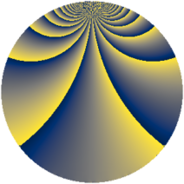# Properties

 Label 230.2.jLevel $230$ Weight $2$ Character orbit 230.j Rep. character $\chi_{230}(9,\cdot)$ Character field $\Q(\zeta_{22})$ Dimension $120$ Newform subspaces $1$ Sturm bound $72$ Trace bound $0$

# Related objects

## Defining parameters

 Level: $$N$$ $$=$$ $$230 = 2 \cdot 5 \cdot 23$$ Weight: $$k$$ $$=$$ $$2$$ Character orbit: $$[\chi]$$ $$=$$ 230.j (of order $$22$$ and degree $$10$$) Character conductor: $$\operatorname{cond}(\chi)$$ $$=$$ $$115$$ Character field: $$\Q(\zeta_{22})$$ Newform subspaces: $$1$$ Sturm bound: $$72$$ Trace bound: $$0$$

## Dimensions

The following table gives the dimensions of various subspaces of $$M_{2}(230, [\chi])$$.

Total New Old
Modular forms 400 120 280
Cusp forms 320 120 200
Eisenstein series 80 0 80

## Trace form

 $$120 q + 12 q^{4} - 4 q^{6} + 8 q^{9} + O(q^{10})$$ $$120 q + 12 q^{4} - 4 q^{6} + 8 q^{9} + 8 q^{11} - 6 q^{15} - 12 q^{16} - 16 q^{19} - 22 q^{20} + 4 q^{24} - 52 q^{25} - 4 q^{26} - 8 q^{29} - 44 q^{30} + 12 q^{31} + 16 q^{35} - 8 q^{36} - 36 q^{39} - 28 q^{41} - 8 q^{44} + 16 q^{45} - 4 q^{46} - 58 q^{49} + 12 q^{50} - 24 q^{51} - 6 q^{54} - 36 q^{55} + 22 q^{56} - 102 q^{59} - 38 q^{60} + 72 q^{61} + 12 q^{64} - 138 q^{65} + 80 q^{66} - 212 q^{69} - 108 q^{70} + 176 q^{71} - 88 q^{74} - 100 q^{75} + 16 q^{76} - 104 q^{79} - 22 q^{80} - 28 q^{81} - 22 q^{84} + 2 q^{85} + 62 q^{86} + 48 q^{89} + 24 q^{90} - 56 q^{91} + 24 q^{94} + 18 q^{95} - 4 q^{96} + 188 q^{99} + O(q^{100})$$

## Decomposition of $$S_{2}^{\mathrm{new}}(230, [\chi])$$ into newform subspaces

Label Dim $A$ Field CM Traces $q$-expansion
$a_{2}$ $a_{3}$ $a_{5}$ $a_{7}$
230.2.j.a $120$ $1.837$ None $$0$$ $$0$$ $$0$$ $$0$$

## Decomposition of $$S_{2}^{\mathrm{old}}(230, [\chi])$$ into lower level spaces

$$S_{2}^{\mathrm{old}}(230, [\chi]) \cong$$ $$S_{2}^{\mathrm{new}}(115, [\chi])$$$$^{\oplus 2}$$